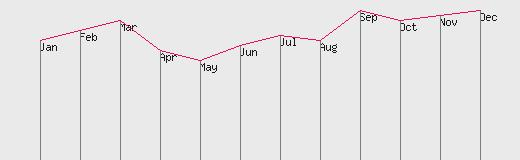# Plotting vertical grid to line graph

We can draw vertical grid to the graph we developed in the basic line graph tutorial. To draw a vertical grid line from each point we have to draw line from graph point to the X axis. Note that the X-axis value from starting and ending point of the grid does not change as the lines are vertical. So x1 and x2 value ( starting and end point ) of x axis is same. The Y axis value starts from the graph point ( y value of the month ) to zero line of our graph and that is the point where Y value is maximum ( the horizontal line at the bottom ). So Y will vary from graph point to \$y_max setting of the image. So to draw the vertical grid line we have to add one line only.
``imageline (\$im,\$x2, \$y_max,\$x2,\$y2,\$grid_color);``
Of course we have to set the value of \$grid_color before this line. We will get image like this. For a better clarity than previous tutorial on basic of line graph we have reduced the height of the graph.The two lines added here are marked in bold letters.
``````<?php
include "db.php";

\$qt=mysql_query("select * from gd_graph");

\$x_gap=40; // The gap between each point in y axis

\$x_max=\$x_gap*13; // Maximum width of the graph or horizontal axis
\$y_max=160; // Maximum hight of the graph or vertical axis
// Above two variables will be used to create a canvas of the image//

\$im = @ImageCreate (\$x_max, \$y_max);
or die ("Cannot Initialize new GD image stream");
\$background_color = ImageColorAllocate (\$im, 234, 234, 234);
\$text_color = ImageColorAllocate (\$im, 233, 14, 91);
\$graph_color = ImageColorAllocate (\$im,25,25,25);

\$grid_color = ImageColorAllocate (\$im,125,125,125);

\$x1=0;
\$y1=0;
\$first_one="yes";
while(\$nt=mysql_fetch_array(\$qt)){
//echo "\$nt[month], \$nt[sales]";
\$x2=\$x1+\$x_gap; // Shifting in X axis
\$y2=\$y_max-\$nt[sales]; // Coordinate of Y axis
ImageString(\$im,2,\$x2,\$y2,\$nt[month],\$graph_color);
// Drawing the grid vertically//
imageline (\$im,\$x2, \$y_max,\$x2,\$y2,\$grid_color);

//Line above is to print month names on the graph
if(\$first_one=="no"){ // this is to prevent from starting \$x1= and \$y1=0
imageline (\$im,\$x1, \$y1,\$x2,\$y2,\$text_color); // Drawing the line between two points//
}
\$x1=\$x2; // Storing the value for next draw
\$y1=\$y2;
\$first_one="no"; // Now flag is set to allow the drawing
}

ImageJPEG (\$im);
?>``````
Line Chart Different types of graphs by using Google ChartsSubscribe to our YouTube Channel here

## Subscribe

* indicates required
Subscribe to plus2netplus2net.com

GD functions in PHP for handling images & graphics

How to check GD support in PHP
Header for an image in browser
Drawing of graphic lines using GD
Plotting line graph by drawing data from table
Adding vertical grid to line graph
Adding rectangles using imagerectangle()
Imagearc drawing arc and circle
Imagefilledarc drawing filled arc and circle
Resizing and adding border to Images
Script for Watermarking image on the fly using PHP GD
Generate image by using user input form data
 adsf 03-11-2014 thanks you for usefull subjects

Post your comments , suggestion , error , requirements etc here